### IMO Shortlist 2017 problem N2

Kvaliteta:
Avg: 0,0
Težina:
Avg: 6,0

Let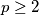$p \geq 2$ be a prime number. Eduardo and Fernando play the following game making moves alternately: in each move, the current player chooses an index$i$ in the set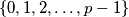$\{0,1,2,\ldots, p-1 \}$ that was not chosen before by either of the two players and then chooses an element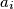$a_i$ from the set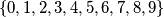$\{0,1,2,3,4,5,6,7,8,9\}$. Eduardo has the first move. The game ends after all the indices have been chosen .Then the following number is computed: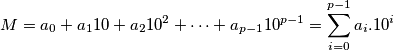. The goal of Eduardo is to make$M$ divisible by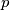$p$, and the goal of Fernando is to prevent this.

Prove that Eduardo has a winning strategy.

Izvor: https://www.imo-official.org/problems/IMO2017SL.pdf